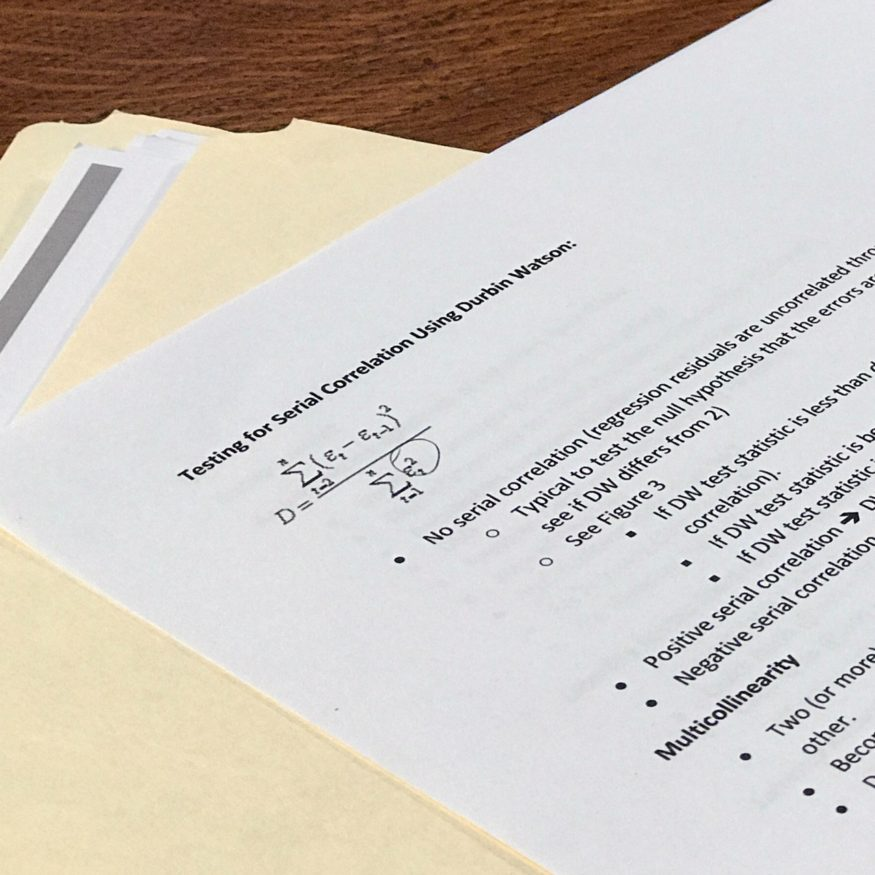# Series 7 Formulas: When Memorization Can’t Be AvoidedThe Series 7 formulas worth knowing range in topics from the Margin section to Debt Securities. We can’t memorize these formulas for you, but we can at least tell you where to start. In today’s post we discuss what causes a lot of problems for many of our students: formulas.

###### So Much Math, So Little Time

We have yet to find a truly great resource for the formulas of the Series 7 Top-Off exam. The truth is, there are quite a lot of formulas to memorize. Most of our students don’t have much more than two months (sometimes at the most!) to prepare for the exam. This is not a lot of time. It can seem especially daunting to those that haven’t used much math in their careers up to this point. The Series 7 Top-Off difficulty level is significantly higher than the SIE Exam difficulty. The significant math component is one key reason why. Although we don’t have a precise figure, we estimate that about 25% of the exam comprises math questions (give or take a little).

###### Series 7 Formulas Worth Knowing

We’ll admit it. We aren’t rocket scientists. Fortunately, you don’t need to be. There are several formulas worth knowing, and many come from Margin and Options sections.

First, let’s take a look at Margin. The most important formulas in the Series 7 Margin section involve calculating equity.

For Long Equity:

Equity = Long Market Value – Debit Balance

For Short Equity:

Equity = Credit Balance – Short Market Value

Both of these are quite necessary when you get into more complicate questions around maintenance margin, SMA, and even accounting for accrued interest on the debit balance. The equity formulas are fundamental, and a key stepping stone toward harder problems.

Next, when it comes to Options consider remembering key Series 7 formulas such as max gain, and max loss for spreads. The same formula can be applied to either one, you just have to know where to apply it. For example:

If you are long 1 ABC Mar 45 call for 5 and short 1 ABC Jun 50 call for 2 then the max gain/loss formulas are as follows:

Max gain = (Strike Price of Option 1 – Strike Price of Option 2) – (Premium of Option 1 – Premium of Option 2)

Max loss = (Premium of Option 1 – Premium of Option 2)

*You should note that the max loss and max gain formulas are swapped when dealing with a credit spread (Hint: the above is a debit spread).

###### Other Important Formulas

Other important Series 7 formulas can be found with regards to mutual funds (sales charge percentages), convertible bonds (conversion ratios), and municipal bonds (amortization/accretion of bond prices). However, one of the most troubling sections for many of our students include the Fundamental Analysis section. This section can be challenging for anyone without any previous accounting background. You don’t need to be a CFA Charterholder to understand it, but even basic accounting courses will help here. The reason being, that on top of having to learn many formulas (e.g., current ratio, quick ratio, working capital, etc.), you also need to know how the financial statements flow (which requires its own formulas: Assets – Liabilities = Shareholders Equity). This section can have its challenges so start with it early.

At Professional Exam Tutoring we recommend starting your studying with the Options sections, Margin and then Fundamental Analysis. All three of these require some formula memorization. There is a lot to cover in those, so leave yourself plenty of time. Most importantly, check out our video courses (or request a tutor) if you need help. With a little effort, anyone can pass this exam. Good luck!